# Strain-controlled criticality governs the nonlinear mechanics of fibre networks

## Abstract

Disordered fibrous networks are ubiquitous in nature as major structural components of living cells and tissues. The mechanical stability of networks generally depends on the degree of connectivity: only when the average number of connections between nodes exceeds the isostatic threshold are networks stable1. On increasing the connectivity through this point, such networks undergo a mechanical phase transition from a floppy to a rigid phase. However, even sub-isostatic networks become rigid when subjected to sufficiently large deformations. To study this strain-controlled transition, we perform a combination of computational modelling of fibre networks and experiments on networks of type I collagen fibres, which are crucial for the integrity of biological tissues. We show theoretically that the development of rigidity is characterized by a strain-controlled continuous phase transition with signatures of criticality. Our experiments demonstrate mechanical properties consistent with our model, including the predicted critical exponents. We show that the nonlinear mechanics of collagen networks can be quantitatively captured by the predictions of scaling theory for the strain-controlled critical behaviour over a wide range of network concentrations and strains up to failure of the material.

## Main

As shown by Maxwell, networks with only central-force interactions exhibit a transition from a floppy to rigid phase at the isostatic point, where the local coordination number, or connectivity 〈z〉 equals the threshold value of 〈z〉 = 2d in d dimensions1. At this point, the number of degrees of freedom is just balanced by the number of constraints, and the system is marginally stable to small deformations. The jamming transition2,3,4 in granular materials and rigidity percolation5,6,7 in disordered spring networks are examples of such a transition. An important feature of these systems is the order of the transition. Jamming exhibits signatures of both first- and second-order transitions, with discontinuous behaviour of the bulk modulus and continuous variation of the shear modulus4,8,9. For networks of springs or fibres, the transition from floppy to rigid is a continuous phase transition, in both bulk and shear moduli4,5,10,11,12,13.

Interestingly, the open fibrous meshworks that provide structure and stability to cells and tissues usually have connectivity below the central-force isostatic point. Extracellular matrices of collagen are a good example of this: the local connectivity is between 3, corresponding to one fibre branching to form two, and 4, for a binary crosslink between distinct fibres. Typical collagen connectivity is measured to be about 3.4, which places such networks below both 2D and 3D isostatic thresholds14,15. Such sub-isostatic networks can, nevertheless, become rigid as a result of other mechanical constraints, such as fibre bending12,16,17, or when subjected to external strain18. The threshold strain, at which the transition occurs, depends on the nature of the applied deformation (shear or tensile) and on the average connectivity of the network, in particular, and other properties of its structure13. However, the order of this strain-induced transition remains unclear.

Here, we study the transition from floppy to rigid states of disordered sub-isostatic networks under simple shear. We show that these networks exhibit a line of second-order transitions (see Fig. 1) at a strain threshold γc(z), for connectivities z well below the isostatic threshold. Moreover, we demonstrate critical behaviour along this line, specifically in the scaling properties of the mechanics, as well as finite-size effects that reflect the underlying divergent correlation length. To test the relevance of these predictions for real materials, we perform experiments on reconstituted networks of collagen, the most prevalent protein in mammals and the mechanical basis of most tissues19. Although collagen has been widely studied for many years, the mechanical properties of collagen matrices remain poorly understood. We find that collagen networks show evidence of critical behaviour in their mechanical response to strain. Strikingly, the measured shear modulus of these networks is in quantitative agreement with the critical behaviour of our model, including the predicted non-mean-field critical exponents.Figure 1: At zero strain, networks undergo a continuous transition from floppy to rigid at the isostatic threshold z = zc.

We study computational models (see Methods) of fibre networks, based on both 2D and 3D lattice-based structures20,21 and Mikado networks in 2D (refs 16,17,22). All networks are, by construction, sub-isostatic and floppy in the absence of bending interactions12. The filaments have a stretching modulus, μ, and bending modulus, κ. These parameters define a dimensionless rigidity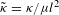, where l is the lattice spacing (mesh size) in lattice-based (Mikado) networks. For a network of elastic fibres,is independent of fibre diameter and is proportional to the network volume fraction, and thus protein concentration (Supplementary Information). The networks are subjected to simple shear strain γ and allowed to relax by minimization of the total elastic energy per unit volume,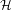, which is calculated using a discrete form of the extensible wormlike chain Hamiltonian (see Methods). The stress and stiffness, in units of μ/ld−1, are obtained fromby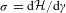and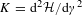, respectively.

In Fig. 2, we show the network stiffness K versus strain γ of a triangular network with 〈z 3.2 for different values of. As sketched in Fig. 1, these networks are characterized by a continuous transition at a strain threshold γc, which is indicated in Fig. 2a by the vertical dashed red line, above which the stiffness K increases continuously from zero for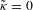. This curve is approached for systems with finite but decreasing, as can be seen by the lower sets of black data points. This second-order phase behaviour is qualitatively analogous to the onset of ferromagnetism on lowering the temperature below the Curie temperature, where the addition of a magnetic field results in finite magnetization in the paramagnetic phase. More precisely, an additional energy10 such as the elastic bending stiffness of fibres12 with a finite coupling constant κ can stabilize otherwise floppy networks. As we show below, the stabilizing effect of κ can be used to reveal the critical behaviour for sub-isostatic systems with z < zc at strains γ γc(z).Figure 2: Computationally obtained stiffness of sub-isostatic networks as a function of the applied strain.

In the absence of the stabilizing effect of bending—that is, for—the continuous nature of the transition in γ is apparent (Supplementary Fig. 2) in the critical scaling of the network stiffness K γ|f in the regime where Δγ = γγc > 0. For γ < γc, the effect of stabilization by bending leads to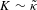. These regimes can be summarized by the scaling form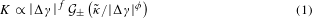where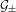is a scaling function, with the positive and negative branches corresponding to Δγ > 0 and Δγ < 0, respectively. This scaling is analogous to that for the conductivity of random resistor networks and fibre networks as a function of connectivity z (refs 10,12,23), although the transition here occurs as a function of γ rather than z, and Δγ represents the distance from the critical line in Fig. 1. In Fig. 2b, we test this scaling relation by plotting Kγ|f versus, according to equation (1). For x 1,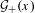is approximately constant and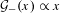. Because K must be finite at Δγ = 0, we also expect K κf/φμ1−f/φ, as one observes from the critical branch in Fig. 2b, consistent with equation (1). To show the generality of this result, we also show in Fig. 2b the corresponding data obtained from Mikado networks, as well as fcc-based 3D lattices. Strikingly, the data collapse with similar exponents f = 0.8 ± 0.05 and φ = 2.1 ± 0.1. The average connectivity for the three different networks is chosen to be in the range 3.2–3.6, comparable to typical biopolymer networks such as collagen14.

To test these predictions for a biologically relevant system, we prepared homogeneous networks of collagen type I at 37 °C and a range of concentrations (Supplementary Fig. 6). We also measured the average coordination number of these networks, z = 3.3 ± 0.1, consistent both with previous study14 and the values used above in our model. Figure 3a shows a series of measurements of the stiffness of these networks as a function of strain for concentrations between 0.7 and 5.0 mg ml−1. Assuming that collagen fibres behave as athermal, homogeneous elastic rods, our model accounts for fibril thickness, mesh size and concentration through the parameter, which is predicted to increase with protein concentration c (see Fig. 3a and Supplementary Information)24. By rescaling our experimental K by the concentration we can compare with the predicted collapse in Fig. 2b. In both experiment and simulation, we obtained the critical strain γc as the inflection point of the logK versus logγ curves (Supplementary Fig. 1a). This strain also coincides with the strain at which the non-affine fluctuations in the network diverge (Supplementary Fig. 1b). On the basis of these considerations, our model predicts that the experimental stiffness K should be governed by the scaling relation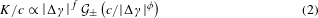In Fig. 3b, we test this prediction by plotting Kγ|f/c versus cγ|φ. We find an excellent collapse with our predictions for 3D networks shown in Fig. 2b, assuming exponents f = 0.8 and φ = 2.1. Moreover, experiments on collagen networks prepared at T = 30 °C are also consistent with our model predictions (Supplementary Fig. 4), implying the generality of our observations of criticality.Figure 3: Experimentally measured stiffness of collagen networks prepared at different concentrations as a function of the applied strain.

In the limit of γ → 0, using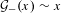(equation (1)), we obtain a scaling relation for the linear modulus and the concentration: K/c c(fφ). Because γc is expected to depend on the average connectivity, which may vary with concentration, we expect γc to show a possible concentration dependence. In fact, as seen in the inset of Fig. 3b, the experimental γc does show a weak γc c−0.14 dependence on the concentration. Moreover, this dependence is consistent with the observed difference between the experimental and theoretical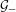branches of Figs 2b and 3b: K/c c1.2 is consistent with K/c c(fφ) and the exponents f and φ in Fig. 3b, as γc c0.2/(fφ) c−0.14.

In contrast to previous empirical rescaling rheology by characteristic, sample-dependent values of stress and strain25,26, our observation of criticality allows us to determine an analytic expression for the nonlinear mechanics of collagen networks over the entire strain range in terms of the scaling function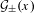. As for ferromagnetic systems, one can obtain an approximate scaling function by numerical inversion of the equation of state27 (Supplementary Information), which in our case is given by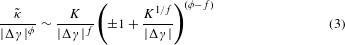Here, ± corresponds to the two branches of the scaling function. In Fig. 3a, we show the predicted K versus γ according to equation (3), withas a fit parameter (Supplementary Information). As noted above, γc is not a free parameter, as it is determined from the inflection point of the experimental stiffening curves. The predictions are in excellent agreement with the experimental data over the entire strain range and for all concentrations.

We further test the critical behaviour by performing finite-size scaling, which is sensitive to the divergence of the correlation length. The modulus should follow the scaling relation (Supplementary Information)where W is the system size and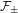is a scaling function, with the positive and negative branches corresponding to Δγ > 0 and Δγ < 0, respectively. In Fig. 4, we plot KWf/ν versus |Δγ| W1/ν, showing consistency with equation (4). These data were obtained for 2D lattice-based networks with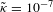. We obtain a good collapse of the data for f = 0.75 ± 0.05 and ν = 2.0 ± 0.1. The lower branch,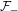, does not vanish as W, owing to the small, finite value of. We confirm, however, thatfor W 1 decreases towards zero as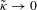(Supplementary Fig. 3).Figure 4: Demonstration of the continuous transition of elasticity in a sub-isostatic network.

In Table 1 we summarize the various values of f and f/φ for different network structures in either 2D or 3D. Although the theoretical results above were chosen to correspond to connectivities 〈z〉 close to the experimental values for collagen networks, we also studied two very different networks—a disordered honeycomb lattice in 2D with 〈z〉 close to 2 (see Fig. 2b inset) and a disordered fcc lattice with 〈z〉 close to the isostatic point (Supplementary Fig. 6). Importantly, the near-isostatic case of the fcc lattice at 〈z 5 exhibits a value f/φ 1/2, consistent with the K κ0.5 scaling reported in ref. 12 for an isostatic network. We note, however, that the individual exponents f and φ here are only defined for sub-isostatic networks, and thus are not expected to coincide with studies of isostatic systems10,12.

In contrast to most previous work on critical phenomena for jamming4, rigidity percolation5 and near-isostatic fibre networks10,12, as well as predictions of topological boundary modes in isostatic lattices28, our focus here has been on networks well below the isostatic point. This situation is particularly relevant to biology, which abounds with structural networks of biopolymers with connectivity between 3 and 4, well below isostaticity in 3D. A central challenge in understanding such systems has been their nonlinear mechanical response. Recently, a Landau-type theory for the nonlinear elasticity of biopolymer gels was proposed using an order parameter describing induced nematic order of fibres in the gel29. Our findings show that the nonlinear mechanics of networks of stiff fibres such as collagen can now be understood quantitatively in terms of critical phenomena associated with a second-order line of transitions below the isostatic point, as indicated in Fig. 1. Both stress and strain can be used as control variables to study the mechanics of fibre networks. In ref. 15, stress was used as control variable to study mechanics of collagen networks. As a floppy network below the point of marginal stability cannot sustain finite stress, however, the criticality we observe here is most naturally studied as a function of strain. Our observations of critical phenomena in collagen networks may provide an explanation for the size-dependent rheology reported in ref. 30. It is plausible that the correlation length associated with criticality can become comparable to the length scale in rheology, leading to finite-size effects. Importantly, as we show, there is a line of critical points that extends over a wide range of network connectivities, covering the physiologically relevant range of 3 < z < 4 in 3D (Supplementary Fig. 8). Moreover, although the linear modulus of collagen networks may be finite in this range owing to the stabilizing influence of bending, the nonlinear response can be quantitatively captured by the scaling functions in equations (1) and (2).

## Methods

### Network generation.

We model lattice-based networks in 2D and 3D, as well as off-lattice (Mikado) networks in 2D. In our lattice-based networks, fibres are arranged on a triangular lattice (2D) or a face-centred cubic (fcc) lattice (3D) of linear dimension W. In 2D, we randomly select two of the three fibres at each vertex, on which we form a binary crosslink—that is, enforcing local 4-fold connectivity of the network in which the third fibre does not interact with the other two. In 3D, where there are six fibres crossing at a point, we randomly connect three separate pairs of fibres at each vertex with binary crosslinks to enforce local 4-fold connectivity. In both 2D and 3D, the average connectivity is further reduced below 4 by random dilution of bonds with a probability (1 − pbond). To generate 3D networks with connectivity exceeding 4, we simply perform random dilution of bonds on a full fcc lattice, with an initial connectivity of 12, until the desired connectivity is reached. The stretching modulus μ and the lattice constant l0 are set to 1 in 2D and 3D. Mikado networks are generated by random deposition of filaments in a 2D box of size W. A freely hinged crosslink is inserted at every point of intersection. The deposition continues until the desired average connectivity is obtained.

### Fibre elasticity.

When a network is subjected to deformation, every fibre undergoes a fractional change in length dl/ds and local curvature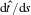, where s denotes the position along the undistorted fibre contour. The elastic energy stored in the fibre is given by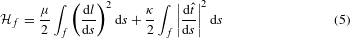where the parameters μ and κ describe the 1D Young’s (stretch) modulus and bending modulus. The integration is evaluated along the fibre contour. The energy stored in a single fibre is calculated by discretizing the equation above31.The total energy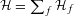is a sum over all fibres, which is minimized under the constraint of the globally applied strain to obtain the energy density in the network.

### Collagen rheology.

For our experiments, rat-tail collagen type I (BD Biosciences) was polymerized at T = 30 °C and T = 37 °C in a physiological buffer solution composed of DMEM1x solution (diluted from 10 ×, Sigma) containing 50 mM HEPES, 1.5 mg ml−1 sodium bicarbonate, 1% FBS (Gibco) and 0.1% antibiotics (pen/strep, Gibco) at pH 7.3. Rheology was performed on a stress-controlled rheometer (Physica MCR 501; Anton Paar) with a 40 mm cone-plate geometry having an 1° cone angle. A solvent trap was added to maintain a humid atmosphere. After 6 h polymerization, stiffness versus stress curves were obtained using a differential protocol. Stiffness curves obtained using a cone-plate of 40 mm and 2° cone angle (not shown) are highly similar to those presented in the main text. More details can be found in the Supplementary Information.

## References

1. 1

Maxwell, J. C. On the calculation of the equilibrium and stiffness of frames. Phil. Mag. 27, 294–299 (1864).

2. 2

Cates, M. E., Wittmer, J. P., Bouchaud, J. P. & Claudin, P. Jamming, force chains, and fragile matter. Phys. Rev. Lett. 81, 1841–1844 (1998).

3. 3

Liu, A. J. & Nagel, S. R. Nonlinear dynamics: jamming is not just cool any more. Nature 396, 21–22 (1998).

4. 4

Van Hecke, M. Jamming of soft particles: geometry, mechanics, scaling and isostaticity. J. Phys. Condens. Matter 22, 033101 (2010).

5. 5

Thorpe, M. F. Continuous deformations in random networks. J. Non-Cryst. Solids 57, 355–370 (1983).

6. 6

Jacobs, D. J. & Thorpe, M. F. Generic rigidity percolation: the pebble game. Phys. Rev. Lett. 75, 4051–4054 (1995).

7. 7

Latva-Kokko, M., Mäkinen, J. & Timonen, J. Rigidity transition in two-dimensional random fiber networks. Phys. Rev. E 63, 046113 (2001).

8. 8

Olsson, P. & Teitel, S. Critical scaling of shear viscosity at the jamming transition. Phys. Rev. Lett. 99, 178001 (2007).

9. 9

Head, D. A. Critical scaling and aging in cooling systems near the jamming transition. Phys. Rev. Lett. 102, 138001 (2009).

10. 10

Wyart, M., Liang, H., Kabla, A. & Mahadevan, L. Elasticity of floppy and stiff random networks. Phys. Rev. Lett. 101, 215501 (2008).

11. 11

Ellenbroek, W. G., Zeravcic, Z., van Saarloos, W. & van Hecke, M. Non-affine response: jammed packings versus spring networks. Europhys. Lett. 87, 34004 (2009).

12. 12

Broedersz, C. P., Mao, X., Lubensky, T. C. & MacKintosh, F. C. Criticality and isostaticity in fibre networks. Nature Phys. 7, 983–988 (2011).

13. 13

Sheinman, M., Broedersz, C. P. & MacKintosh, F. C. Nonlinear effective-medium theory of disordered spring networks. Phys. Rev. E 85, 021801 (2012).

14. 14

Lindström, S. B., Vader, D. A., Kulachenko, A. & Weitz, D. A. Biopolymer network geometries: characterization, regeneration, and elastic properties. Phys. Rev. E 82, 051905 (2010).

15. 15

Licup, A. J. et al. Stress controls the mechanics of collagen networks. Proc. Natl Acad. Sci. USA 112, 9573–9578 (2015).

16. 16

Head, D. A., Levine, A. J. & MacKintosh, F. C. Deformation of crosslinked semiflexible polymer networks. Phys. Rev. Lett. 91, 108102 (2003).

17. 17

Wilhelm, J. & Frey, E. Elasticity of stiff polymer networks. Phys. Rev. Lett. 91, 108103 (2003).

18. 18

Alexander, S. Amorphous solids: their structure, lattice dynamics and elasticity. Phys. Rep. 296, 65–236 (1998).

19. 19

Fratzl, P. Collagen: Structure and Mechanics (Springer Science & Business Media, 2008).

20. 20

Broedersz, C. P. & MacKintosh, F. C. Molecular motors stiffen non-affine semiflexible polymer networks. Soft Matter 7, 3186–3191 (2011).

21. 21

Broedersz, C. P., Sheinman, M. & MacKintosh, F. C. Filament-length-controlled elasticity in 3d fiber networks. Phys. Rev. Lett. 108, 078102 (2012).

22. 22

Conti, E. & MacKintosh, F. C. Cross-linked networks of stiff filaments exhibit negative normal stress. Phys. Rev. Lett. 102, 088102 (2009).

23. 23

Straley, J. P. Critical phenomena in resistor networks. J. Phys. C 9, 783–795 (1976).

24. 24

Achilli, M. & Mantovani, D. Tailoring mechanical properties of collagen-based scaffolds for vascular tissue engineering: the effects of ph, temperature and ionic strength on gelation. Polymers 2, 664–680 (2010).

25. 25

Motte, S. & Kaufman, L. J. Strain stiffening in collagen I networks. Biopolymers 99, 35–46 (2013).

26. 26

Arevalo, R. C., Kumar, P., Urbach, J. S. & Blair, D. L. Stress heterogeneities in sheared type-I collagen networks revealed by boundary stress microscopy. PLoS ONE 10, e011802 (2015).

27. 27

Arrott, A. & Noakes, J. E. Approximate equation of state for nickel near its critical temperature. Phys. Rev. Lett. 19, 786–789 (1967).

28. 28

Kane, C. & Lubensky, T. Topological boundary modes in isostatic lattices. Nature Phys. 10, 39–45 (2014).

29. 29

Feng, J., Levine, H., Mao, X. & Sander, L. M. Alignment and nonlinear elasticity in biopolymer gels. Phys. Rev. E 91, 042710 (2015).

30. 30

Arevalo, R. C., Urbach, J. S. & Blair, D. L. Size-dependent rheology of type-I collagen networks. Biophys. J. 99, L65–L67 (2010).

31. 31

Head, D. A., Levine, A. J. & MacKintosh, F. C. Distinct regimes of elastic response and deformation modes of cross-linked cytoskeletal and semiflexible polymer networks. Phys. Rev. E 68, 061907 (2003).

## Acknowledgements

We thank M. Vahabi for useful discussions. This work was carried out on the Dutch national e-infrastructure with the support of SURF Cooperative. This work is part of the research programme of the Foundation for Fundamental Research on Matter (FOM), which is financially supported by the Netherlands Organisation for Scientific Research (NWO). This work is further supported by NanoNextNL, a micro and nanotechnology programme of the Dutch Government and 130 partners.

## Author information

Authors

### Contributions

A.S., A.J.L. and K.A.J. contributed equally to the work. A.S., A.J.L., R.R., M.S. and F.C.M. conceived and developed the model and simulations. A.S., A.J.L., R.R. and M.S. performed the simulations. K.A.J. and G.H.K. designed the experiments. K.A.J. performed the experiments. All authors contributed to the writing of the paper.

### Corresponding authors

Correspondence to G. H. Koenderink or F. C. MacKintosh.

## Ethics declarations

### Competing interests

The authors declare no competing financial interests.

## Supplementary information

### Supplementary information

Supplementary information (PDF 1950 kb)

## Rights and permissions

Reprints and Permissions

• ### Intracellular nonequilibrium fluctuating stresses indicate how nonlinear cellular mechanical properties adapt to microenvironmental rigidity

• Ming-Tzo Wei
• , Sabrina S. Jedlicka
•  & H. Daniel Ou-Yang

Scientific Reports (2020)

• ### Bioinspired hydrogel-based nanofluidic ionic diodes: nano-confined network tuning and ion transport regulation

• Congcong Zhu
• , Yunfei Teng
• , Ganhua Xie
• , Pei Li
• , Yongchao Qian
• , Bo Niu
• , Pei Liu
• , Weipeng Chen
• , Xiang-Yu Kong
• , Lei Jiang
•  & Liping Wen

Chemical Communications (2020)

• ### Loops versus lines and the compression stiffening of cells

• M. C. Gandikota
• , Katarzyna Pogoda
• , Anne van Oosten
• , T. A. Engstrom
• , A. E. Patteson
• , P. A. Janmey
•  & J. M. Schwarz

Soft Matter (2020)

• ### Connectivity and plasticity determine collagen network fracture

• Federica Burla
• , Simone Dussi
• , Cristina Martinez-Torres
• , Justin Tauber
• , Jasper van der Gucht
•  & Gijsje H. Koenderink

Proceedings of the National Academy of Sciences (2020)

• ### The physics of the mouthfeel of caviar and other fish roe

• Thomas A. Vilgis

International Journal of Gastronomy and Food Science (2020)